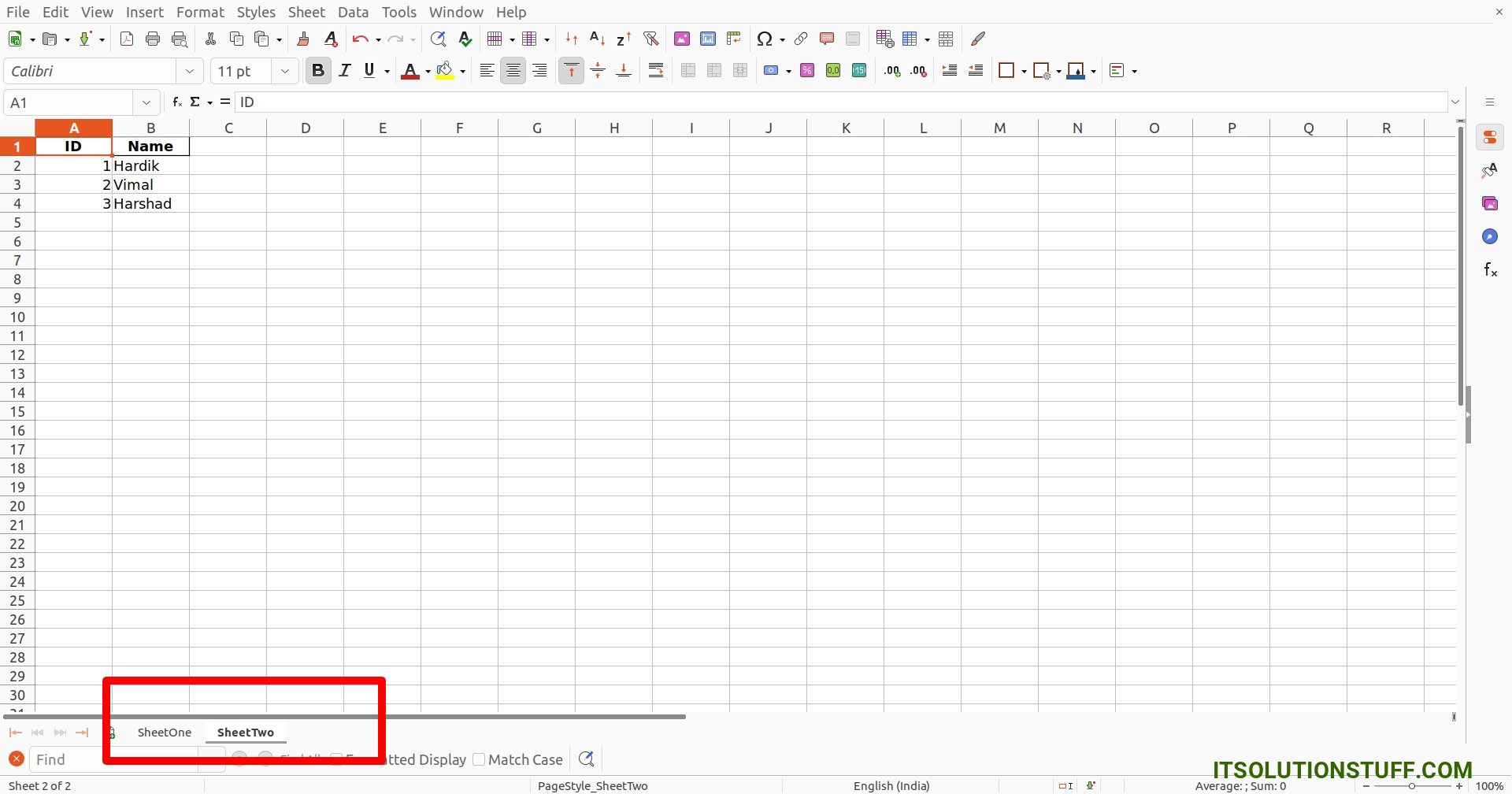# Python Pandas Create Excel File Example

By Hardik Savani July 29, 2023 Category : Python

Hello Dev,

This extensive guide will teach you python pandas create excel file example. you will learn how to create excel file in python pandas. I explained simply how to create excel file from dataframe in python. This example will help you how to create xlsx file in python pandas. Alright, letâ€™s dive into the steps.

Pandas is an open-source Python package. Pandas is used to analyze data. We can work with DataFrame using Python Pandas.

I will give you simple two examples of creating an excel file using pandas. in the first example, we will create a simple xlsx file with one sheet. in the second example, we will create xlsx file with multiple sheets. so let's see the below examples.

You can use these examples with python3 (Python 3) version.

If you haven't install pandas in your system then you can install using the below command:

```pip install pandas
```

Example 1:

main.py

```import pandas as pd

# Create DataFrame for XLSX File
df = pd.DataFrame({'ID': [1, 2, 3],
'Name': ["Hardik Savani", "Vimal Kashiyani", "Harshad Pathak"],

# Create a Pandas Excel writer using XlsxWriter
writer = pd.ExcelWriter('demo.xlsx', engine='xlsxwriter')

# Convert the dataframe to an XlsxWriter Excel object.
df.to_excel(writer, sheet_name='Sheet1', index=False)

# Save Data to File
writer.save()
```

Output:

Now, It will generated demo.xlsx file in your root path with the below content.

demo.xlsxExample 2: Multiple Sheet

main.py

```import pandas as pd

# Create a Pandas Excel writer using XlsxWriter
writer = pd.ExcelWriter('demo.xlsx', engine='xlsxwriter')

# Create SheetOne with Data
df = pd.DataFrame({'ID': [1, 2, 3],
'Name': ["Hardik Savani", "Vimal Kashiyani", "Harshad Pathak"],

df.to_excel(writer, sheet_name='SheetOne', index=False)

# Create SheetTwo with Data
df = pd.DataFrame({'ID': [1, 2, 3],

df.to_excel(writer, sheet_name='SheetTwo', index=False)

# Save Data to File
writer.save()
```

Output:

Now, It will generated demo.xlsx file in your root path with the below content.

demo.xlsx